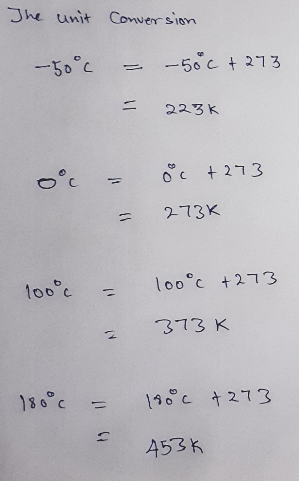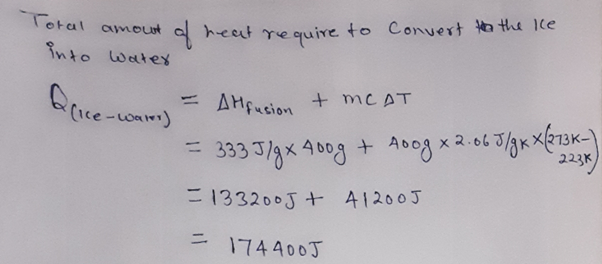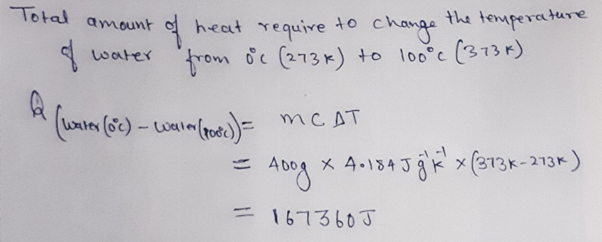# Calculate the energy needed to convert 400. g of ice at -50.0 °C to steam at 180.0 °C. The heat of fusion of water is 333 J/g, and the heat of vaporization is 2256 J/g. The specific heat of ice is 2.06 J/g∙K, and the specific heat of liquid water is 4.184 J/g∙K. The specific heat of steam is 1.86 J/g∙K.J

Question
1 views

Calculate the energy needed to convert 400. g of ice at -50.0 °C to steam at 180.0 °C. The heat of fusion of water is 333 J/g, and the heat of vaporization is 2256 J/g. The specific heat of ice is 2.06 J/g∙K, and the specific heat of liquid water is 4.184 J/g∙K. The specific heat of steam is 1.86 J/g∙K.

J

check_circle

Step 1Step 2Step 3...

### Want to see the full answer?

See Solution

#### Want to see this answer and more?

Solutions are written by subject experts who are available 24/7. Questions are typically answered within 1 hour.*

See Solution
*Response times may vary by subject and question.
Tagged in